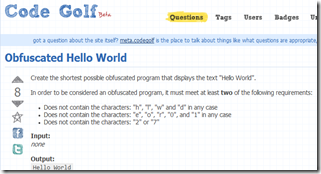using System;

# Obfuscated JS Hello World CodeGolf

Finally the codegolf Stackexchange site is in beta. So I decided to give it a try, as I found this nice codegolf:So…. Behold my solution with JavaScript:

``````    javascript:(_=(_=[][(f=!!(_='')+_)+(b=({}+_))+(\$=(c=(d=!_+_))+d)])())[f+'l'+(a=d)+\$]("H"+a+'ll'+b+' W'+b+c+'ld');
``````

Feel free to try it out. Just paste it in your browser’s address bar and let it do the magic.

Well if you would like to know how it works I suggest you read this blog post first (I’ve actually shared it in my Google Reader feed a month back, so you might have already seen it). The script uses the same principle to acquire the sort() function (and consequently the window object and alert() function) as described there. I also found this nice video that was very helpful.